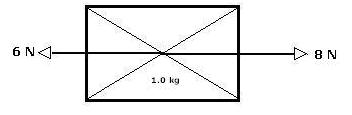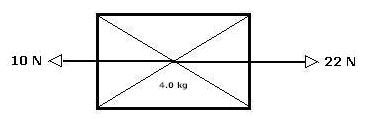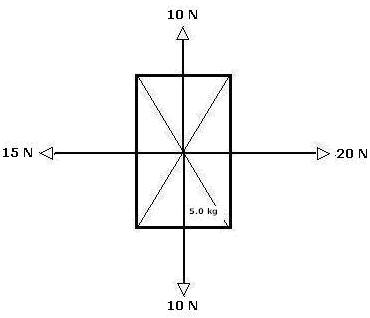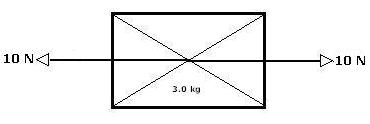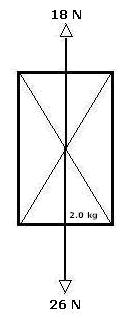Based upon the free-body diagrams below, calculate Fnet and the acceleration of each rectangular block: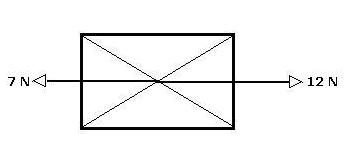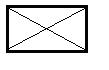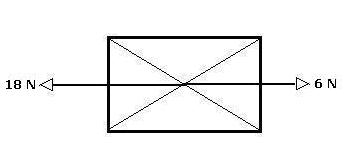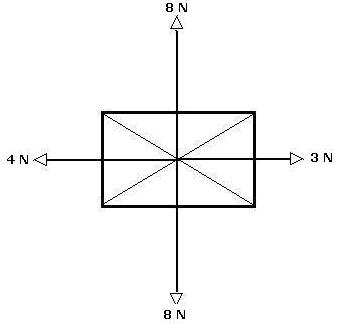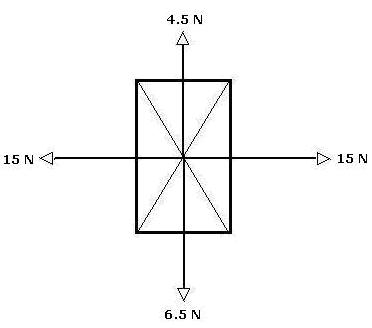The Concept of Net Force
and
Free-Body Diagrams

Calculate the net force acting on each rectangular body at left and sketch a vector representing the net force acting on the rectangular body on the figure to the right: